### Home > CALC > Chapter 4 > Lesson 4.4.3 > Problem4-156

4-156.
1. For the following functions, find an end behavior function. Explain your method. Homework Help ✎

1.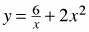2.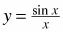3.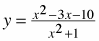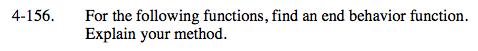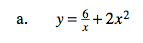$\text{Consider }\lim_{x\rightarrow \infty }\frac{6}{x}+2x^{2}.$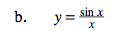If a function has a horizontal asymptote, then that is the end behavior.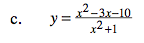If a function has a horizontal asymptote, then that is the end behavior. You can find a horizontal asymptote by evaluating